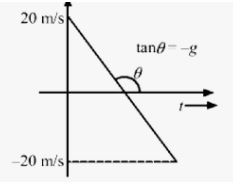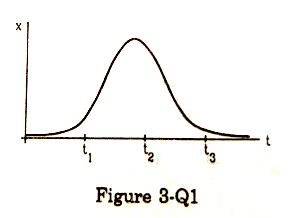Friday, March 24, 2023

# Rest and Motion Kinematics HC Verma Solutions Que for Short Ans Ch-3

Rest and Motion Kinematics HC Verma Solutions Que for Short Ans Ch-3 Vol-1 Class-11. Step by step Solutions of HC Verma Ch-3 Rest and Motion Kinematics  Que for Short Ans for Class-11 Volume- 1 (Concept of Physics). Visit official Website for detail information about ISC Board Class-11 Physics.

## Rest and Motion Kinematics HC Verma Solutions Que for Short Ans Ch-3 Vol-1 Class-11

 Board ISC and other board Publications Bharti Bhawan Publishers Ch-3 Rest and Motion Kinematics Class 11 Vol 1st writer H C Verma Book Name Concept of Physics Topics Solution of Questions for short answer Page-Number 48, 49

-: Select Topics :-

Objective-I

Objective-II

Exercise

### Rest and Motion Kinematics HC Verma Solutions Concepts of Physics Ch-3 Vol-1 Class-11

(page-48)

#### Question-1

Galileo was punished by the Church for teaching that the sun is stationary and the earth moves around it. His opponents held the view that the earth is stationary and the sun moves around it. If the absolute motion has no meaning, are the two viewpoints not equally correct or equally wrong?

The absolute motion has no meaning. In the relative motion view, the two viewpoints are the same. Hence, both viewpoints are equally correct or equally wrong.

#### Question-2

When a particle moves with constant velocity, its average velocity, its instantaneous velocity and its speed are all equal. Comment on this statement.

Constant velocity means that a particle has the same direction and speed at every point. So, its average velocity and instantaneous velocity are equal. Its speed being a scalar quantity is equal in magnitude only.

#### Question-3

A car travels at a speed of 60 km/hr due north and the other at a speed of 60 km/hr due east. Are the velocities equal? If no, which one is greater? If you find any of the questions irrelevant, explain.

No, as the directions of the cars are different, their velocities are not equal, as velocity is a vector quantity.

We cannot compare velocities on the basis of direction only, so the question is irrelevant.

#### Question-4

A ball is thrown vertically upward with a speed of 20 m/s. Draw a graph showing the velocity of the ball as a function of time as it goes up and then comes back.#### Question-5

The velocity of a particle is towards west at an instant. Its acceleration is not towards west, not towards east, not towards north and towards south. Give an example of this type of motion

Motion of a projectile at the highest point of its path: Because at this point, the projectile has only horizontal component of velocity. So, it can have velocity towards west and acceleration in the vertically downward direction.

#### Question-6

At which point on its path a projectile has the smallest speed?

A projectile has the lowest speed at the highest point of its path because the vertical component of velocity is zero at this point.

#### Question-7

Two particles A and B start from rest and move for equal time on a straight line. The particle A has an acceleration a for the first half of the total time and 2a for the second half. The particle B has an acceleration 2a for the first and a for the second half. Which particle has covered larger distance?

## =3.5 at²

Question-8

If a particle is accelerating, it is either speeding up or speeding down. Do you agree with this statement?

Acceleration does not mean speeding up or speeding down. It means the change of velocity either in direction or in magnitude.

#### Question-9

A food packet is dropped from a plane going at an altitude of 100 m. What is the path of the packet as seen from the plane? What is the path as seen from the ground ? If someone asks “what is the actual path”, what will you answer?

The path of the packet (as seen from the plane) is a vertically downward straight line, as the horizontal velocity of the packet and the plane is the same.
As seen from the ground, the path of the packet is a parabola.
The path is defined with respect to some reference frame. As there is no absolute reference frame, no actual path is defined.

#### Question-10

Give example where

(a) the velocity of a particle is zero but its acceleration is not zero.

(b)  the velocity is opposite in direction to the acceleration.

(c)  the velocity is perpendicular to the acceleration.

(a) At the highest point when a particle is thrown vertically upwards.

(b) While going up when a particle is thrown vertically upwards.

(c)  At the highest point of a full projectile.

#### Question-11

In figure shows the x coordinate of a particle as a function of time. Find the sings of vand ax at t = t1, t = t2 and t = t3.Slope of the x–t graph gives the velocity and change in the slope gives the acceleration.
At t = t1,
Slope = Positive ⇒ Velocity = Positive
Slope is increasing ⇒ Acceleration = Positive At t = t2,
Slope = Constant ⇒ Velocity = Zero
Slope is constant ⇒ Acceleration = Negative At t = t3,
Slope = Negative ⇒ Velocity = Negative
Slope is increasing ⇒ Acceleration = Negative

(page-49)

#### Question-12

A player hits a baseball at some angle. The ball goes high up in space. The player runs and catches the ball before it hits the ground. Which of the two (the player or the ball) has greater displacement?

The displacement of the ball and the player is the same, as the initial and final points are the same.

#### Question-13

The increase in the speed of a car is proportional to the additional petrol put into the engine. Is it possible to accelerate a car without putting more petrol or less petrol into the engine?

Yes, it is possible to accelerate a car without putting more petrol or less petrol in the engine. This can be done by driving the car on a circular or curved track at a uniform speed.

#### Question-14

Rain is falling vertically. A man running on the road keeps his umbrella tilted but a man standing on the street keeps his umbrella vertical to protect himself from the rain. But both of them keep their umbrella vertical to avoid the vertical sun-rays. Explain.

The speed of rain is less, so as we run, the direction of the relative velocity of the rain changes. But as the speed of light is very high, there is a measurable change in the relative velocity of light w.r.t. a person due to the relative motion between the sunrays and the person.

—: End of Rest and Motion Kinematics HC Verma Solutions Que for Short Ans   :–

Thanks

RELATED ARTICLES